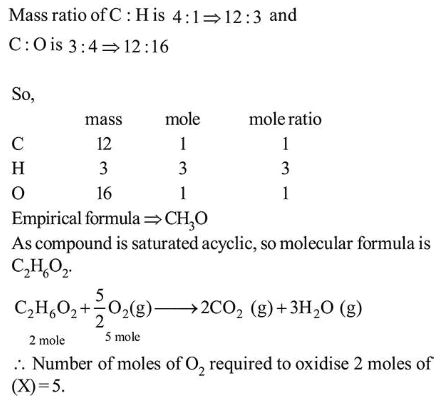# The ratio of the mass percentages of 'Question:

The ratio of the mass percentages of ' $\mathrm{C} \& \mathrm{H}$ ' and ' $\mathrm{C} \& \mathrm{O}$ ' of a saturated acyclic organic compound ' $\mathrm{X}$ ' are $4: 1$ and $3: 4$ respectively. Then, the moles of oxygen gas required for complete combustion of two moles of organic compound ' $X$ ' is ____________ .

Solution:

(5)